# AP Physics 1 Multiple-select Practice Test 4

### Test Information9 questions18 minutes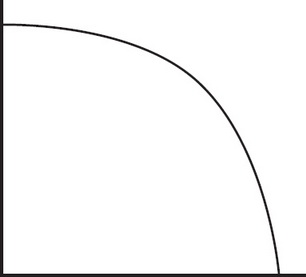1. The graph above could be a representation of which two of the following situations? Select two answers.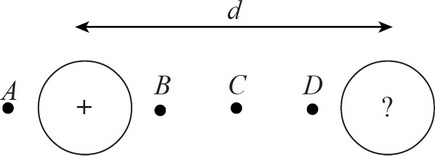2. Two spheres are separated by a distance d. The first sphere has a known positive charge. The second sphere has a charge of a magnitude greater than the magnitude of the charge on the first sphere but an unknown sign. Given this information, at which two of the above locations could a positive test charge potentially experience 0 net electrical force? Select two answers.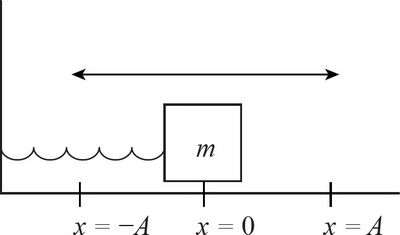3. A spring-block system with a block of known mass m is oscillating under ideal conditions. Which two of the following pieces of additional sets of information would allow you to calculate the amplitude of the block's motion? Select two answers.

4. An object traveling at x m/s can stop at a distance d m with a maximum negative acceleration. If the car is traveling at 2x m/s, which of the following statements are true? Select two answers.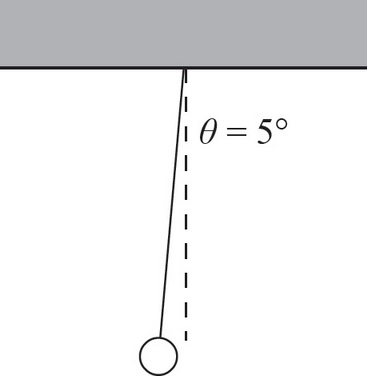5. A 2 kg mass is attached to a massless, 0.5 m string and is used as a simple pendulum by extending it to an angle θ = 5° and allowing it to oscillate. Which of the following changes will change the period of the pendulum? Select two answers.

6. N resistors (N > 2) are connected in parallel with a battery of voltage Vo. If one of the resistors is removed from the circuit, which of the following quantities will decrease? Select two answers.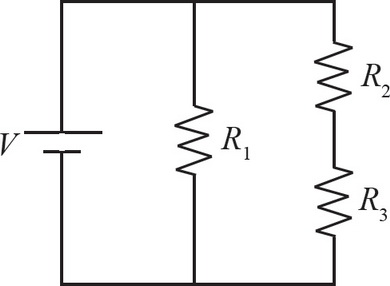7. Which of the following will decrease the current through R3 in the circuit above? Select two answers.

8. A sound wave travels through a metal rod with wavelength λ and frequency f. Which of the following are true? Select two answers.

9. If vector A has components (5, 3), for which of the following vectors B would the magnitude of the vector sum of A + B be equal to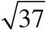? Select two answers.